Next: Summary of Plane Up: No Title Previous: Example 2: Synthetic

# Computing Plane Projective Transformations

Estimate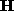for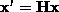,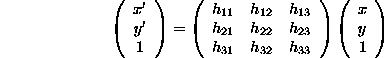where = is equality up to scale. Minimum number of correspondences: 4 points (non-collinear) or 4 lines (non-concurrent).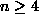correspondences

n = 4 will be covered as a special case. Each point correspondence generates two linear equations for the elements of(dividing by the third component to remove the unknown scale factor)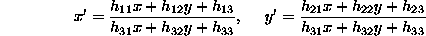and multiplying out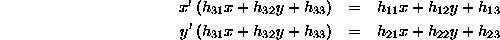Thenpoints generates 2 n linear equations, which are sufficient to solve for. The problem with projective transformations is that the matrixis only recovered up to scale.

Homogeneous Solutions There are two methods of dealing with the unknown scale factor in a homogeneous matrix

1. Choose one of the matrix elements to have a certain value. For example,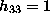.
2. Solve for the matrix up to scale.

Method I

If, then the above equations can be written: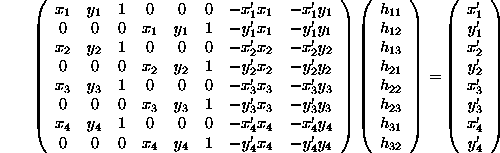for 4 points. A linear solution is then obtained, in the usual manner, by solving the set of linear simultaneous equations. Similarly, for n > 4 points, a solution can be obtained using a pseudo-inverse.

The problem with this approach is that if the true solution is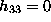, then this cannot be reached. Consequently, a poor quality estimate ofwill be obtained.

Method II

The equations,can be rearranged as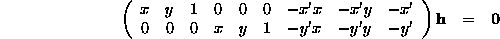where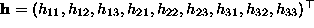is the matrixwritten as a vector. For 4 points,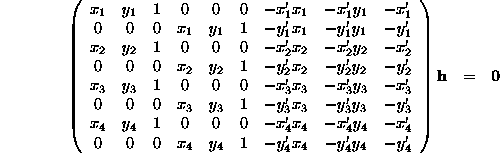which has the form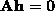, with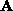a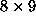matrix. The solution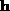is the (one dimensional) kernel of.

For n > 4 real point correspondences,is a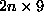matrix, and there will not be a solution to. In this case, a sensible procedure is to again minimise the residuals. It can be shown that the vector that minimises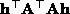subject to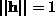, is the eigenvector with least eigenvector of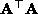. In the case of n = 4 the least eigenvalue is zero, and the eigenvector is the kernel of.Next: Summary of Plane Up: No Title Previous: Example 2: Synthetic

Bob Fisher
Wed Apr 16 00:58:54 BST 1997